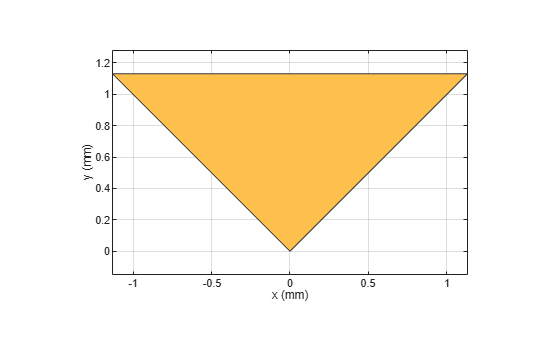delta

Create delta shape

Description

Use delta object to create a delta shape on the X-Y plane.

Creation

Description

example

deltashape = delta creates a delta shape on the X-Y plane.

deltashape = delta(Name=Value) sets Properties using one or more name-value arguments. For example, delta(ReferencePoint=[1 1]) creates a delta shape with the reference point at [1 1]. Properties not specified retain their default values.

Properties

expand all

Name of the delta shape, specified as a character vector or string scalar.

Example: deltashape = delta(Name='deltaShape')

Data Types: char

Reference point of delta shape in Cartesian coordinates, specified as a two-element vector of nonnegative elements. Use the reference point to modify the shape relative to its initial position.

Example: deltashape = delta(ReferencePoint=[1 1])

Data Types: double

Outer radius of the delta, specified as a positive scalar in meters.

Data Types: double

Inner radius of the delta, specified as a nonnegative scalar in meters. This value truncates the delta from the tip.

Data Types: double

Angel of the delta shape, specified as a positive scalar in degrees. The value of the angle must be greater than 0 degrees and lesser than 180 degrees.

Example: shape = delta(Angle=50)

Data Types: double

Object Functions

 add Boolean unite operation on two RF PCB shapes and Shape1 & Shape2 for RF PCB shapes area Calculate area of RF PCB shape in square meters intersect Boolean intersection operation on two RF PCB shapes mesh Change and view mesh properties of metal or dielectric in PCB component minus Shape1 - Shape2 for RF PCB shapes plus Shape1 + Shape2 for RF PCB shapes rotate Rotate RF PCB shape about defined axis rotateX Rotate RF PCB shape about x-axis rotateY Rotate RF PCB shape about y-axis and angle rotateZ Rotate RF PCB shape about z-axis subtract Boolean subtraction operation on two RF PCB shapes scale Change size of RF PCB shape by fixed amount show Display PCB component structure or PCB shape translate Move RF PCB shape to new location

Examples

collapse all

Create a delta shape with default properties.

deltashape = delta
deltashape =
delta with properties:

Name: 'mydelta'
ReferencePoint: [0 0]
Angle: 90

View the shape.

show(deltashape)